Practical Geostatistics 2000 Read the reviews Answers to the Exercises ErrataContact Isobel Clark with new errata or errors

We would like to thank everyone who provided feedback.

Errata from First Edition and First reprint of PG2000 may be found on the original errata pageNew: Pierre Mousset-Jones at University of Nevada Reno, Mackay School of Mines tells me his students have found that I made a total pig’s ear of the calculations for Question 7 in Chapter 4 Lognormal examples. Mea Culpa. To much to put in here. Find Pages 128-130 in pdf form here. Large number of corrections courtesy of Kathy Flanders at Auburn University in Alabama. Remember that these are only potential answers not divinely inspired solutions!! Remember also that hand calculated answers will differ (sometimes significantly) from answers shown in screen dumps from software. In Chapter 2, especially, where we are dividing statistics by ‘n’ instead of ‘n-1’ answers can be very different, depending on the size of ‘n’ (obviously?). Less than that and it is probably calculator, PC or spreadsheet precision.

Preface

Notation

Chapter 1 Introduction

Chapter 2 Why a statistical approach?

Chapter 3 Normal distributions

Page 48: calculation of confidence for the standard deviation at the top of page should read: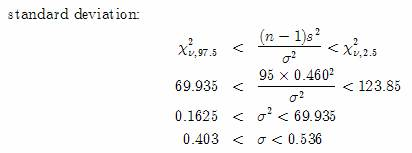Page 49: Last paragraph at bottom of page should read:  That is, our best estimate for μ is 16.941 %Ash and we can be 95% confident that the true mean lies between 16.345 and 17.537 %Ash”

Page 58: half way down the page, calculation should be: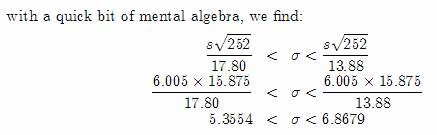Page 85: the two lines below the figure for Q11 should read:

“We will continue the exercise on the (erroneous) assumption of Normality. For a central 95% confidence interval on the true unknown population mean:”

Chapter 4 Lognormal distributions (and others)

Page 120: tables at the top of the page should look like this: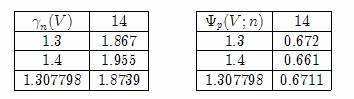Page 120: Fourth paragraph of text should read “………. has a mean of zero and a standard deviation of 1, ………….”

Chapter 5 Discrete distributions

Page 154: the final answer to question 3, should be: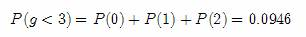Chapter 6 Hypothesis testing

There appears to be some confusion between the subscripts on the sample statistics and those on the degrees of freedom for the F test. It is necessary to divide the larger variance by the smaller to use Table 5(a) and (b). However, degrees of freedom ν1 refers to the degrees of freedom attached to the top line of the ratio not to the first set of samples. Similarly ν2 refers to the degrees of freedom relevant to the bottom line of the ration, not necessarily to the second set of samples. Sorry about this, standard notation!

Page 171: Table of  t values in the centre of the page should read: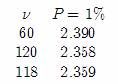Page 173: answer for ‘t’ statistic should be –6.313.

Chapter 7 Relationships

Chapter 8 The Spatial aspect

Page 206: horrific spreadsheet calculation error on Isobel’s part!!! Table and paragraph at the bottom of the page should read: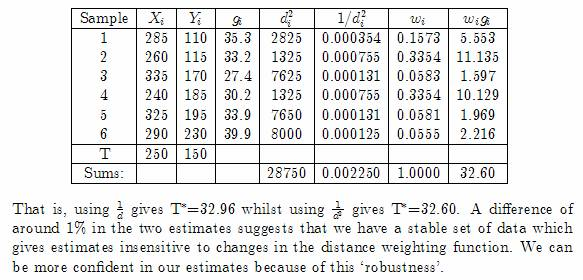Chapter 9 The semi-variogram

Chapter 10 Estimation and Kriging

Chapter 11 Areas and volumes

Chapter 12 Other kriging approaches

Bibliography

Tables

Table 1 has been mislabelled. Headings for probability column should read F not f. Apologies for all confusion.

Index